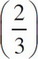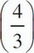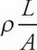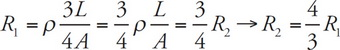# AP Physics 1 Question 47: Answer and Explanation

### Test Information

Question: 47

2. Wire #1 has three times the length and twice the diameter of Wire #2. If both wires are made of the same material and if R1 is the resistance of Wire #1 and R2 is the resistance of Wire #2, then which of the following is true?

• A. R2 =R1
• B. R2 =R1
• C. R2 = 6R1
• D. R2 = 12R1

B The resistance of a wire is determined by R =so let's say R2 =. Because the diameter is doubled for Wire #1, the cross-sectional area is actually multiplied by a factor of 4. So,